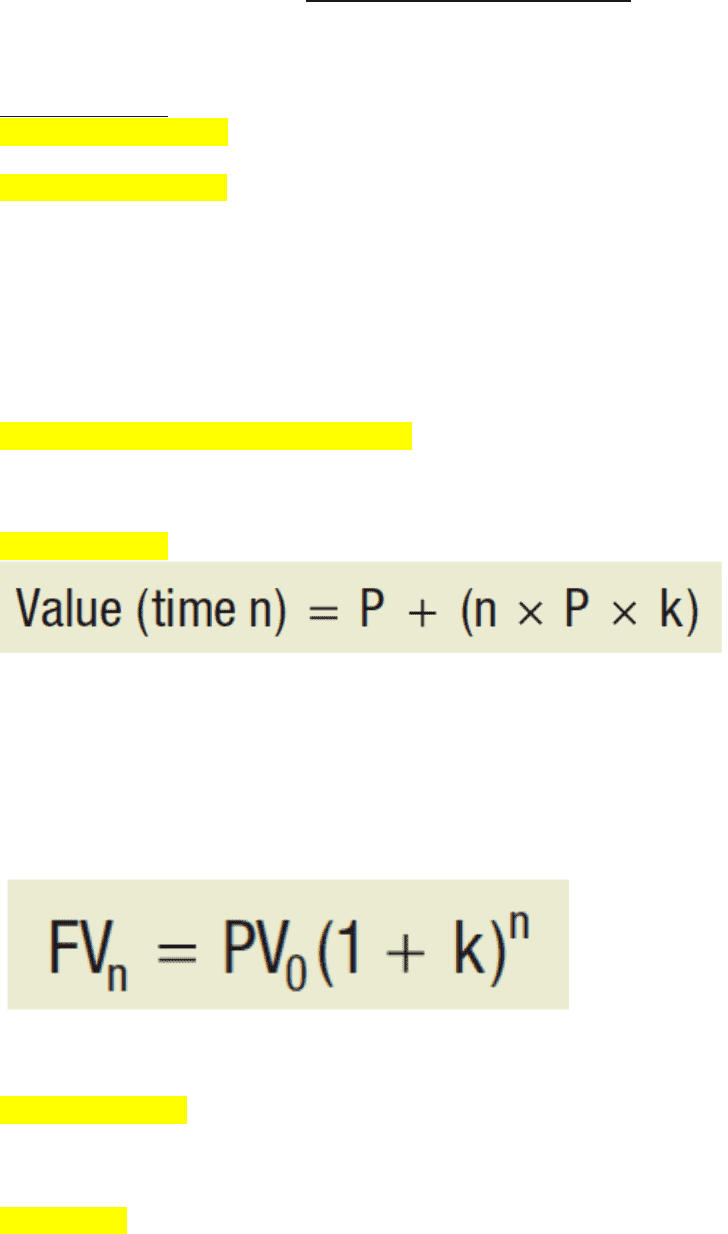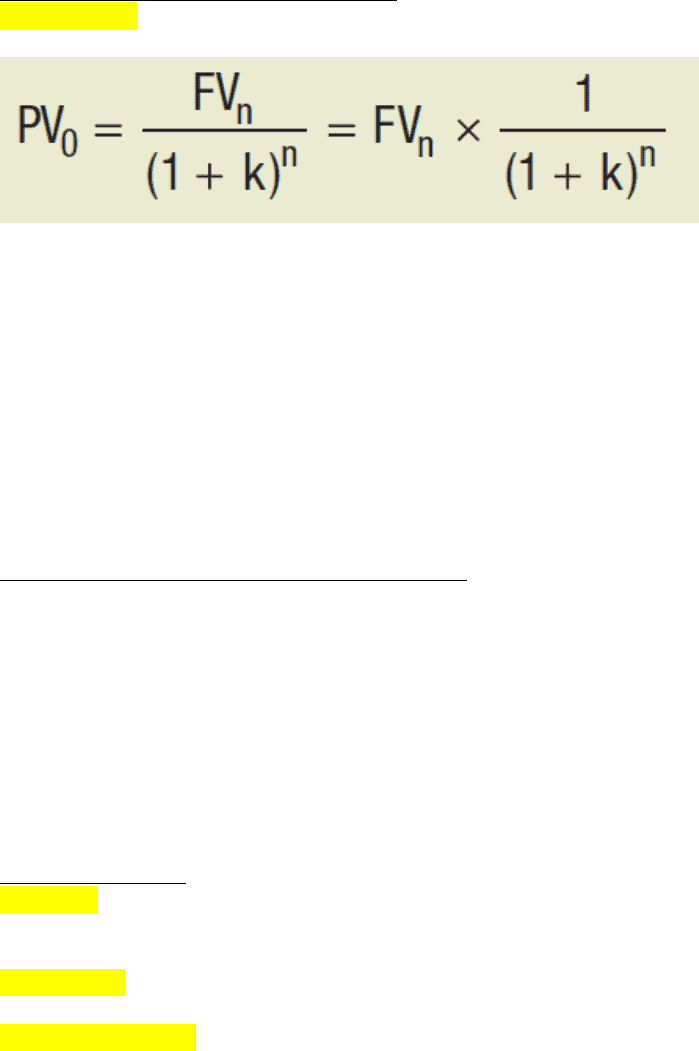Textbook Notes (290,000)
CA (170,000)
UTSG (10,000)
Chapter 5

# RSM230H1 Chapter Notes - Chapter 5: Money Lo, Compound Interest, Accrued Interest

Department
Rotman Commerce
Course Code
RSM230H1
Professor
Ray Daroga
Chapter
5

This preview shows pages 1-2. to view the full 6 pages of the document.Ch. 5: Time Value of Money
LO 5.1 Explain the importance of the time value of money and how it is related to an
investor's opportunity costs
Opportunity Cost
Time value of money: idea that a dollar today is worth more than a dollar in the future
Medium of exchange: something that can be used to facilitate transactions
Money represents our ability to buy goods and services, and has no value in and of itself
Opportunity costs such as investing the dollar to earn a return are what produce the time
value of money
The opportunity cost of money is the interest rate that would be earned by investing it
o Call the interest rate the "price of money"
o Knowing this rate helps us determine the value of money received at different times
Required rate of return (discount rate): the market interest rate (k) or the investor's
opportunity cost
LO 5.2 Define simple interest and explain how it works
Simple interest: interest paid or received on only the initial investment (principal)
P = principal
n = number of periods
P x k = interest
LO 5.3 Define compound interest and explain how it works
Compound interest: interest that is earned on the principal amount invested and on any accrued
interest
The amount of compound interest earned increases every year
FVn = future value at time n
(1 + k)n = future value interest factor (FVIF)
Compound return represents the average annual growth rate in the value of \$1 invested at the
start of the period
Involves the same reinvestment rate assumption as compound interest rate
Often referred to as the geometric return
Basis point: 1/100 of 1%

Only pages 1-2 are available for preview. Some parts have been intentionally blurred.Earning just a few basis points more on one investment causes the future value of the
portfolio to compound much faster, however the search for additional returns often leads
investors to underestimate the associated risks
Discounting (Computing Present Values)
Discounting: finding the present value of a future value by accounting for the time value of
money
1 / (1 + k) n, is called the discount factor or present value interest factor (PVIF)
PVIP = 1/FVIP
The greater the discount rate, the greater the FVIF (and future value) and the smaller the
PVIF (and present value) and vice versa
o E.g low interest rates and pension payments. Pension funds estimate the present value
of future pension payouts to plan members (their liabilities) based on discounting
these future pension payments using current interest rates
As a result of low interest rates, the present value of these pension liabilities
have increased dramatically
Determining Rates of Return or Holding Periods
FVn = PV0 (1 + k)n
Future value problems: How much will I have in n years at x percent if I invest \$y today?
Present value problems: What is the value today of receiving \$z in n years if the interest
rate is x percent?
IRR problems: What rate of return will I earn if I invest \$y today for n years and get \$z?
Period problems: How long do I have to wait to get \$z if I invest \$y today at x percent?
LO 5.4 Differentiate between an ordinary annuity and an annuity due, and explain how
special constant payment problems can be valued as annuities and in special cases, as
perpetuities.
Ordinary Annuities
Annuity: regular payments on an investment that are for the same amount and are paid at the
same interval of time
Ex: Car loan, mortgage payment
Cash flows: actual cash generated from an investment
Ordinary annuity: equal payments that are made at the end of each period of time